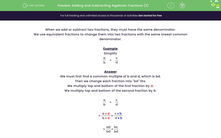# Practise Adding and Subtracting Algebraic Fractions

In this worksheet, students will add or subtract algebraic fractions giving their answer as a single fraction.Key stage:  KS 3

Curriculum topic:   Algebra

Curriculum subtopic:   Use and Interpret Algebraic Notation

Popular topics:   Algebra worksheets

Difficulty level:#### Worksheet Overview

When we add or subtract two fractions, they must have the same denominator.

We use equivalent fractions to change them into two fractions with the same lowest common denominator.

Example

Simplify

 a + c b d

We must first find a common multiple of b and d, which is bd.

Then we change each fraction into 'bd' ths.

We multiply top and bottom of the first fraction by d.

We multiply top and bottom of the second fraction by b.

 a + c b d

 = a x d + c x b b x d d x b

 = ad + bc bd bd

Let's get on to the questions.

### What is EdPlace?

We're your National Curriculum aligned online education content provider helping each child succeed in English, maths and science from year 1 to GCSE. With an EdPlace account you’ll be able to track and measure progress, helping each child achieve their best. We build confidence and attainment by personalising each child’s learning at a level that suits them.

Get started